# Young measure

(diff) ← Older revision | Latest revision (diff) | Newer revision → (diff)

parametrized measure, relaxed control, stochastic kernel

A family of probability measures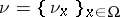, one for each pointin a domain(cf. also Probability measure), associated to a sequence of functionswith the fundamental property that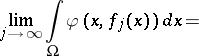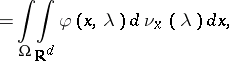for any Carathéodory function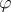. The Young measuredepends upon the sequence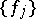but is independent of([a1], [a5], [a8]).

The main area where Young measures have recently been used is optimization theory. Optimization problems where a local, integral cost functional is to be minimized in a suitable class of functions often lack optimal solutions because of the presence of some non-convexity. In such cases, a single function is unable to reproduce the optimal behaviour, due precisely to this lack of optimal solutions, and one must resort to sequences (the so-called minimizing sequences) in order to comprehend the main features of optimality. From the horizon of the above-mentioned class of cost functionals, Young measures furnish a convenient way of dealing with optimal behaviour paying attention only to those features that make a behaviour optimal and disregarding accidental properties.

The way in which this process is accomplished can be described as follows. Let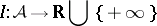be a local, integral cost functional defined on an admissible class of functions. Typically,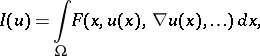whereindicate higher-order derivatives. The optimization problem of interest is to comprehend how the infimum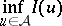is realized. One introduces a generalized optimization problem, intimately connected to the one above, by puttingwhenis the Young measure associated to the sequence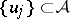. If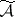stands for the set of all such Young measures, one would like to understand the optimal behaviour for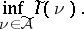Due to the fundamental property of the Young measure indicated above, the optimal behaviour for this new optimization problem can always be described with a single element in, which in turn is generated by minimizing sequences of the original optimization problem. The whole point is being able to study the generalized optimization problem by itself, and then interpret that information in terms of minimizing sequences of the initial optimization problem. The main issue here is to find ways of characterizing the admissible setthat may allow for an independent treatment of the generalized optimization problem. In particular, understanding how constraints inare determined by constraints inis a major challenge. See [a3], [a4].

Young measures were originally introduced in the context of optimal control problems [a9], [a10] (cf. also Optimal control), and have also been used in some situations for problems in partial differential equations [a2], [a6], [a7].

How to Cite This Entry:
Young measure. Encyclopedia of Mathematics. URL: http://encyclopediaofmath.org/index.php?title=Young_measure&oldid=16883
This article was adapted from an original article by Pablo Pedregal (originator), which appeared in Encyclopedia of Mathematics - ISBN 1402006098. See original article